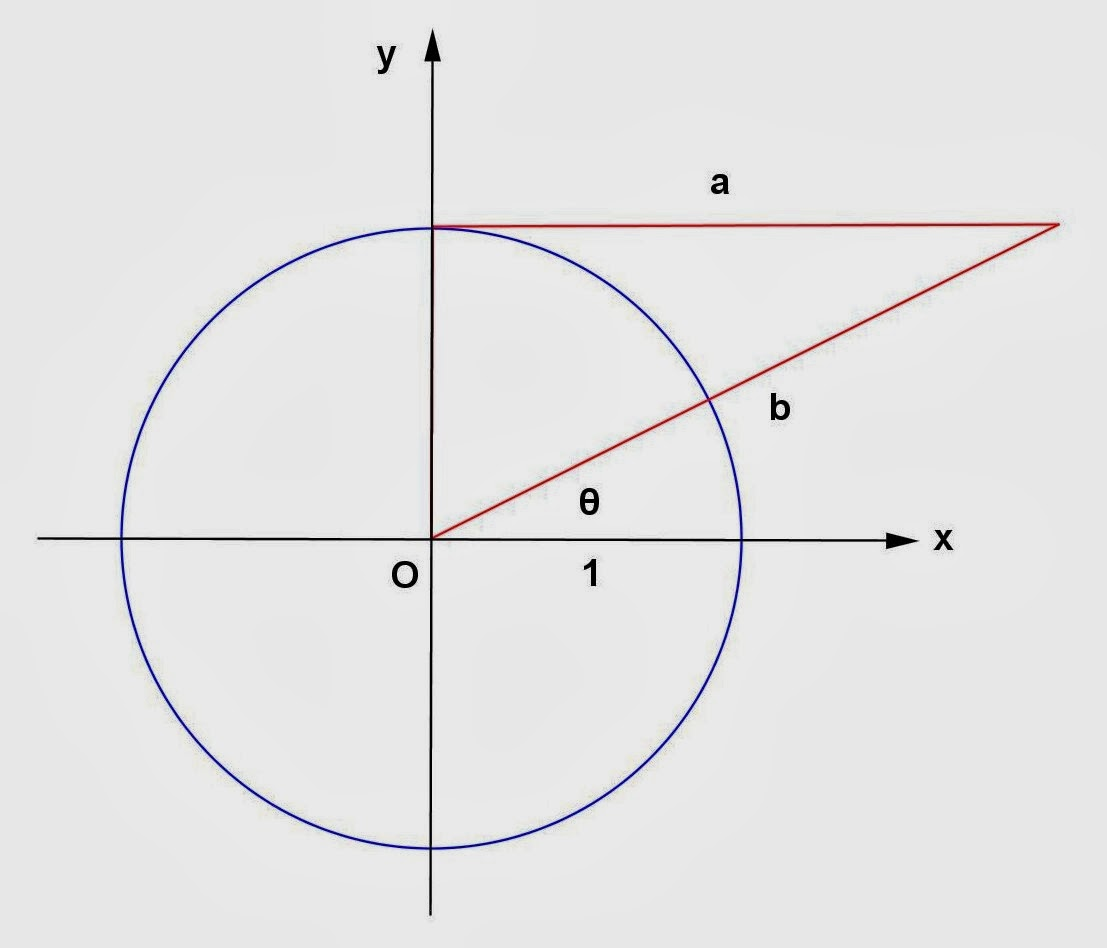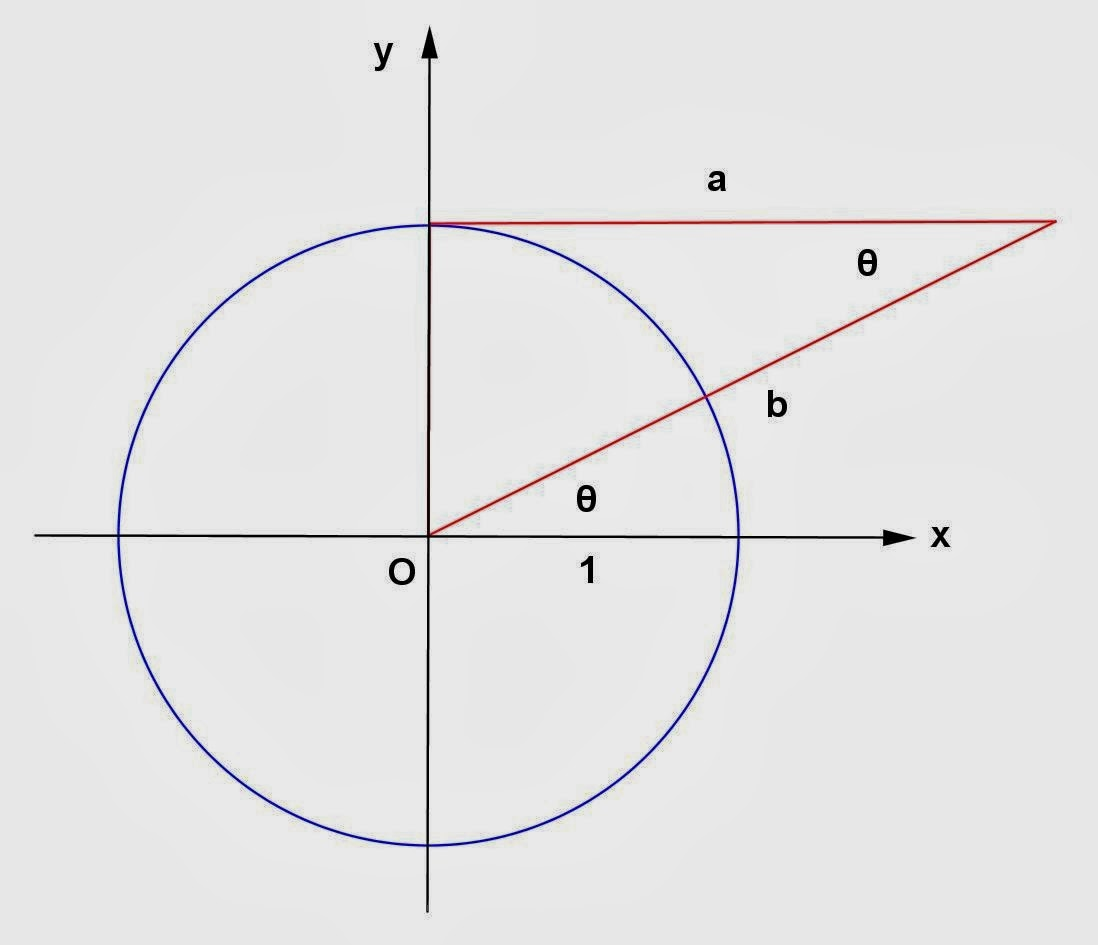## Friday, January 3, 2014

### Circle - Triangle Problems

Category: Trigonometry, Plane Geometry

"Published in Vacaville, California, USA"

Express the lengths a and b in the figure in terms of the trigonometric ratios of θPhoto by Math Principles in Everyday Life

Solution:

Consider the given figure abovePhoto by Math Principles in Everyday Life

The given figure consists of a circle and a triangle. One side of a triangle is equal to the radius of a circle which is 1. The other side of a triangle which is a is tangent to a circle at the y-axis. If a is perpendicular to the other side of a triangle and to the y-axis, then it follows that the given triangle is a right triangle.Photo by Math Principles in Everyday Life

If a is perpendicular to y-axis, then it follows that a is parallel to x-axis. If b is a transversal line that passes thru the parallel lines a and the x-axis, then it follows that the alternating interior angles are congruent. In this case, the angle formed by lines b and the x-axis is congruent to the angle formed by lines a and b which is θ.

Since we know the side and the opposite angle of a right triangle, then we can get the values of a and b in terms of trigonometric functions of θ as follows